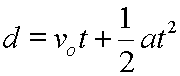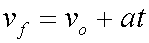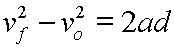# Kinematics, Equations for Accelerated Motion

In introductory mechanics there are three equations that are used to solve kinematics problems:These three equations are explained below. For each equation we will:

• Present an introduction.
• Understand a derivation, or origin.
• Use algebra to rearrange and solve the equation for all of its variables.
• Do some random valued multiple-choice problems.
• Cover some details and additional information.

Symbols used in these equations:

 d Displacement or change in position vo Original velocity, the velocity at the start of the acceleration vf Final velocity, the velocity at the end of the acceleration. a Acceleration, this is a constant acceleration t Time

There are many cross links in the discussions that follow.

So if you need to, you can always jump back to here for a clear starting point in your navigation.

Displacement and AccelerationVelocity and AccelerationTime Independent AccelerationCustom Search